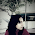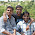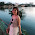## Index

 STA & SI Chapter1 Chapter2 Chapter3 Chapter4 Chapter5 Chapter6 Chapter7 Chapter8 Introduction Static Timing Analysis Clock Advance STA Signal Integrity EDA Tools Timing Models Other Topics

 Extraction & DFM Chapter1 Chapter2 Chapter3 Chapter4 Chapter5 Chapter6 Introduction Parasitic Interconnect Corner (RC Corner) Manufacturing Effects and Their Modeling Dielectric Layer Process Variation Other Topic

## (Effect of Transistor's Size On the Slew)

 STA & SI:: Chapter 2: Static Timing Analysis 2.1 2.2 2.3a 2.3b 2.3c 2.4a Timing Paths Time Borrowing Basic Concept Of Setup-Hold Basic Concept of Setup-Hold Violation Examples:S-H Time/Violation Timing Path Delay 2.4b 2.4c 2.5a 2.5b 2.6a 2.6b Interconnect Delay Models Delay - Wire Load Model Maximum Clock Frequency Calculate “Max Clock Freq”-Examples Fix Setup-Hold Violation-1 Fix Setup-Hold Violation-2 2.6c 2.7a 2.7b 2.7c 2.8 Fix Setup-Hold Violation-3 Incr/Decr Delay Method-1 Incr/Decr Delay Method-2 Incr/Decr Delay Method-3 10 ways to fix Setup-Hold Violation.

Static Timing analysis is divided into several parts:

In the Last post we have discussed - how the wire length effects the slew? Now lets discuss about the effect of size of the transistors. Also before that let's discuss few basics also.

Size of transistor:

There are 2 parameters – Width and Length, by which you can decide the size of the transistor. For a particular technology – Channel - length is almost constant. So it means Width is going to decide the size of the transistor. Below figure will refresh your memory - about which, parameter I am talking.

If you want to increase the width of the transistor, then you have 2 options. One – Just increase the Width directly, Second -connect multiple transistors in parallel in such a way that their effective impact remains same. For example – if you want to manufacture a transistor with a width of 20um and a length of 0.2um then it’s similar (not exactly the same) to having four transistors connected in parallel, each with a width of 5um and a length of 0.2um. Here I am not going to discuss the difference in both the way of representation of Layout. If you are interested then you can check any basic book of CMOS design. Below figure will refresh your memory (Note: Below figure I have copied from www.eda-utilities.com )

Now since we are talking about the transition time /transition delay /slew, we know that it depend on the capacitance and resistance. So before we start to discuss how width (means size of the transistor) impact on the transition delay, we should know what all are the capacitance associated with the transistor. Below diagram help you in that. (Note: Below figure I have copied from www.eda-utilities.com )

How the capacitance are calculated (means whole derivation and explanation), I will discuss some other time, right now I am writing/copying the value of these capacitance directly.

Note: the reference of above formulas is from the book written by “J.P.uyemura” - Cmos Logic Circuit Design Edition -2002.

Now from the above, you can see that Gate Capacitance (this gate capacitance has 3 component – Gate to Base, Gate to Source and Gate to Drain) has dependence on the Width of the Channel (W). So it means, if you increase the width, Gate Capacitance will increase and Vice-Versa.
Source and Drain Capacitance has a multiplying factor As and Ad (which is equavilant to WxLs or WxLd). It means source and drain capacitance also increases with Width of the Channel and Vice-Versa.

Now let’s talk about the Resistance. Below Resistance formula is with respect to NMOS. You can derive similar formula for PMOS also (Just replace subscript “n” with “p” J ).

Now here the Resistance is inversely proportional to Width of the Transistor.

Effect of Device Size on the Slew (transition time) and Propogation Delay.:

I can’t write in a single line the effect of size of transistor on the slew because it’s not straight forward (I know you might have doubt on my statementL). There are some other factors which we have to consider. I hope, below paragraph helps you to understand the same.

Consider the above circuit. Gate “A” is the Driving Gate and Gate “B” is the Driven Gate. If we will expand this with the actual capacitance, it will be something similar to…

• Capacitance Cgd12 is the Gate Capacitance of Driving Gate A due to overlap in M1 and M2.
• Cdb1 and Cdb2 are the diffusion capacitances due to the reverse-biased pn-junction
• Cw is the wiring capacitance (pp, fringe, and interwire) that depends on the length and width of the connecting wire. It is a function of the fanout of the gate and the distance to those gates.
• Cg3 and Cg4 are the gate capacitance of the fanout gate (Driven gate).

If we increase the size of the transistor (Width of the Transistor) it’s current carrying capability increase. Means “larger is the size of a transistor, the larger is the driving capability (the ability to source or sink current) of a transistor”. Thus a larger transistor would normally make its output transition faster (when output load is constant).  The output load of a driving gate consists of the source/drain capacitance of the driving gate, the routing capacitance of wire, and the gate capacitance of the driven gate.
The larger is the output load, the longer is the time to charge or discharge it. This would increase the transition (rise or fall) time and propagation delay.

Let me summarize few important points.
• On increasing the Size of Gate A –
• On Resistance Decreases (R - inversely proportional to W)
• Means large Driving capability (Ability to source or sink current)
• Decrease the time to charge the output load (capacitance) (Consists of  source/drain capacitance of the driving gate, the routing capacitance of wire, and the gate capacitance of the driven gate) **
• Means - Output Transition time of Gate A and Input Transition time for Gate B decreases.

I am sure you have noticed that I have marked point 3 with ** because there are terms and conditions. :)

On increasing the Size of the Gate A – Source/Drain Capacitance also increases which are the part of output load of Gate A. Means it’s going to increase the output load. That means as I have mentioned in my point no 3 – that can be possible only when S/D Capacitance of Driving gate are not dominating the rest of the Capacitance. Which is only possible when either “Net capacitance is large” (length of wire is large) or “Size of the driven gate (Gate B) is large” (which increase the Gate capacitance of GateB) or “Both should be true”.
So for Minimizing Propagation Delay, A fast Gate/Cell is required, which is only possible by
1. Keeping the output capacitance CL small (it decreases the charging and discharging time). And for this
• Minimize the area of drain pn junctions. (Decrease W)
• Minimize Interconnect capacitance. (Decrease wire/net Length)
• Avoid large fan-out. Means Minimize gate capacitance of Driven Cell. (Decrease W of Driven cell)
2. Decreasing the equivalent Resistance of the transistors
• Decrease L (For a particular technology Node It’s almost constant)
• Increase W
• But this increases pn junction area and hence CL.

So if we want to use the size of the transistor as one of the parameter to increase/decrease of the propagation/transition delay, then we should have understanding of the design and also it depends on the property of Driven Cell and Net length also.

Few last points:
1. "Delay reduces with increase in input transition and constant load capacitance".
2. "Delay increases with increase in output capacitance and constant input transition"
• Because on increasing the output capacitance – charging and discharging time will increase.

So we can say that
The delay of cell directly depends on input transition and output capacitance.

In the next post we will discuss about the effect of Threshold voltage of the Transistor on the "Transition Delay" and "Propagation Delay".

1.Good article, Thank you ~

2.This proves that every time increasing the size of the Transistor will not work for fixing setup,slews,etc..

3."Delay reduces with increase in input transition and constant load capacitance"......plz explain this point

1.it means larger is the size of transistor,more faster will be the transition and thus less delay

2.firstly we should understand that what s delay.... u can see that if we increase i/p transition that means inc the set up time n also say we inc the freq. of the clk

4.1.if we decrease W then source drain capacitance of driving cell,and gate capacitance of driven cell decreases but resistance get increase...so which factor we should consider,,,resistance or capacitance?

1.No One can tell this 100%. but yes what ever be dominating - we have to consider their effect.

5.I could not resist myself from commenting after reading this one. This is genius of an article, you are doing a great job. Just keep it coming. And thanks for posts so far.

6.if we increase the drive strength of gate it will decrease the resistance. but it will increase the capacitance effect. so how it is possible to reduce the delay with this capacitance effect

7.This comment has been removed by a blog administrator.

8.Thanks for sharing the information. It is very useful for my future. keep sharing
whatsapp baixar | Facebook Lite

9.If we increase the size of the transistor, diffusion cap will increase. Will that not add to the output load cap and increase the time for charging and hence increase the delay (slew for the driven gate) ?

10.The knowledge you share really changes me in life, I sincerely thank you for the things you have done, sure your blog will help more people. Sincerely thanks

11.Thank you so much for explaining about VLSI concepts.I like your way of explanation.I can easily understand your concepts.Keep in updating.
Software Testing Training in Bangalore
AWS Training in Chennai

12.Thanks for your post! Through your pen I found the problem up interesting! I believe there are many other people who are interested in them just like me! Thanks your shared!... I hope you will continue to have similar posts to share with everyone! I believe a lot of people will be surprised to read this article!
io games

13.14.Interesting article! Thank you for sharing them! I hope you will continue to have similar posts to share with everyone!